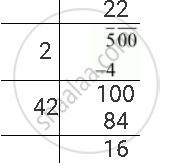Share

# These Are 500 Children in a School. for a P.T. Drill They Have to Stand in Such a Manner that the Number of Rows is Equal to Number of Columns. How Many Children Would Be Left Out in this Arrangement? - CBSE Class 8 - Mathematics

ConceptSquare Roots - Finding Square Root by Division Method

#### Question

These are 500 children in a school. For a P.T. drill they have to stand in such a manner that the number of rows is equal to number of columns. How many children would be left out in this arrangement?

#### Solution

It is given that there are 500 children in the school. They have to stand for a P.T. drill such that the number of rows is equal to the number of columns.

The number of children who will be left out in this arrangement has to be calculated. That is, the number which should be subtracted from 500 to make it a perfect square has to be calculated.

The square root of 500 can be calculated by long division method as follows.The remainder is 16.

It shows that the square of 22 is less than 500 by 16. Therefore, if we subtract 16 from 500, we will obtain a perfect square.

Required perfect square = 500 − 16 = 484

Thus, the number of children who will be left out is 16.

Is there an error in this question or solution?

#### APPEARS IN

NCERT Solution for Mathematics Textbook for Class 8 (2018 to Current)
Chapter 6: Squares and Square Roots
Ex. 6.40 | Q: 9 | Page no. 108
Solution These Are 500 Children in a School. for a P.T. Drill They Have to Stand in Such a Manner that the Number of Rows is Equal to Number of Columns. How Many Children Would Be Left Out in this Arrangement? Concept: Square Roots - Finding Square Root by Division Method.
S